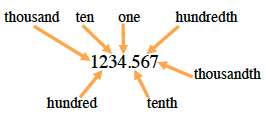### Home > CC1MN > Chapter 6 Unit 6 > Lesson CC1: 6.1.1 > Problem6-11

6-11.

Round each number to the specified place.

Refer to the diagram below if you have trouble remembering the name of each place.1. $198.59$ (ten)

2. $5462.9554$ (thousandth)
$5462.955$
3. $724.82338$ (tenth)
4. $851.392$ (one)
$851$